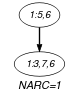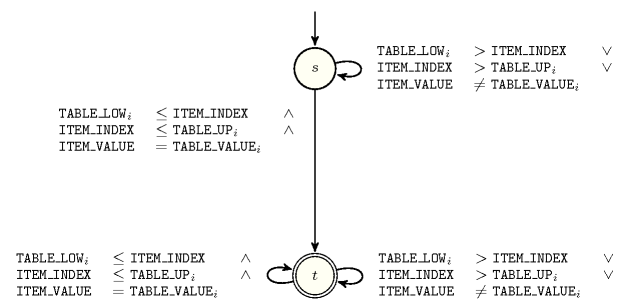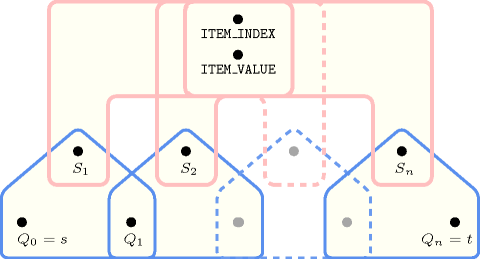## 5.374. stage_element

Origin

Choco, derived from $\mathrm{𝚎𝚕𝚎𝚖𝚎𝚗𝚝}$.

Constraint

$\mathrm{𝚜𝚝𝚊𝚐𝚎}_\mathrm{𝚎𝚕𝚎𝚖𝚎𝚗𝚝}\left(\mathrm{𝙸𝚃𝙴𝙼},\mathrm{𝚃𝙰𝙱𝙻𝙴}\right)$

Usual name

$\mathrm{𝚜𝚝𝚊𝚐𝚎}_\mathrm{𝚎𝚕𝚝}$

Synonym

$\mathrm{𝚜𝚝𝚊𝚐𝚎}_\mathrm{𝚎𝚕𝚎𝚖}$.

Arguments
 $\mathrm{𝙸𝚃𝙴𝙼}$ $\mathrm{𝚌𝚘𝚕𝚕𝚎𝚌𝚝𝚒𝚘𝚗}\left(\mathrm{𝚒𝚗𝚍𝚎𝚡}-\mathrm{𝚍𝚟𝚊𝚛},\mathrm{𝚟𝚊𝚕𝚞𝚎}-\mathrm{𝚍𝚟𝚊𝚛}\right)$ $\mathrm{𝚃𝙰𝙱𝙻𝙴}$ $\mathrm{𝚌𝚘𝚕𝚕𝚎𝚌𝚝𝚒𝚘𝚗}\left(\mathrm{𝚕𝚘𝚠}-\mathrm{𝚒𝚗𝚝},\mathrm{𝚞𝚙}-\mathrm{𝚒𝚗𝚝},\mathrm{𝚟𝚊𝚕𝚞𝚎}-\mathrm{𝚒𝚗𝚝}\right)$
Restrictions
 $\mathrm{𝚛𝚎𝚚𝚞𝚒𝚛𝚎𝚍}$$\left(\mathrm{𝙸𝚃𝙴𝙼},\left[\mathrm{𝚒𝚗𝚍𝚎𝚡},\mathrm{𝚟𝚊𝚕𝚞𝚎}\right]\right)$ $|\mathrm{𝙸𝚃𝙴𝙼}|=1$ $|\mathrm{𝚃𝙰𝙱𝙻𝙴}|>0$ $\mathrm{𝚛𝚎𝚚𝚞𝚒𝚛𝚎𝚍}$$\left(\mathrm{𝚃𝙰𝙱𝙻𝙴},\left[\mathrm{𝚕𝚘𝚠},\mathrm{𝚞𝚙},\mathrm{𝚟𝚊𝚕𝚞𝚎}\right]\right)$ $\mathrm{𝚃𝙰𝙱𝙻𝙴}.\mathrm{𝚕𝚘𝚠}\le \mathrm{𝚃𝙰𝙱𝙻𝙴}.\mathrm{𝚞𝚙}$ $\mathrm{𝚒𝚗𝚌𝚛𝚎𝚊𝚜𝚒𝚗𝚐}_\mathrm{𝚜𝚎𝚚}$$\left(\mathrm{𝚃𝙰𝙱𝙻𝙴},\left[\mathrm{𝚕𝚘𝚠}\right]\right)$
Purpose

Let ${\mathrm{𝚕𝚘𝚠}}_{i}$, ${\mathrm{𝚞𝚙}}_{i}$ and ${\mathrm{𝚟𝚊𝚕𝚞𝚎}}_{i}$ respectively denote the values of the $\mathrm{𝚕𝚘𝚠}$, $\mathrm{𝚞𝚙}$ and $\mathrm{𝚟𝚊𝚕𝚞𝚎}$ attributes of the ${i}^{th}$ item of the $\mathrm{𝚃𝙰𝙱𝙻𝙴}$ collection. First we have that: ${\mathrm{𝚕𝚘𝚠}}_{i}\le {\mathrm{𝚞𝚙}}_{i}$ and ${\mathrm{𝚞𝚙}}_{i}+1={\mathrm{𝚕𝚘𝚠}}_{i+1}$.

Second, the $\mathrm{𝚜𝚝𝚊𝚐𝚎}_\mathrm{𝚎𝚕𝚎𝚖𝚎𝚗𝚝}$ constraint forces the following equivalence:

${\mathrm{𝚕𝚘𝚠}}_{i}\le \mathrm{𝙸𝚃𝙴𝙼}.\mathrm{𝚒𝚗𝚍𝚎𝚡}\wedge \mathrm{𝙸𝚃𝙴𝙼}.\mathrm{𝚒𝚗𝚍𝚎𝚡}\le {\mathrm{𝚞𝚙}}_{i}⇔\mathrm{𝙸𝚃𝙴𝙼}.\mathrm{𝚟𝚊𝚕𝚞𝚎}={\mathrm{𝚟𝚊𝚕𝚞𝚎}}_{i}$.

Example
$\left(\begin{array}{c}〈\mathrm{𝚒𝚗𝚍𝚎𝚡}-5\mathrm{𝚟𝚊𝚕𝚞𝚎}-6〉,\hfill \\ 〈\begin{array}{ccc}\mathrm{𝚕𝚘𝚠}-3\hfill & \mathrm{𝚞𝚙}-7\hfill & \mathrm{𝚟𝚊𝚕𝚞𝚎}-6,\hfill \\ \mathrm{𝚕𝚘𝚠}-8\hfill & \mathrm{𝚞𝚙}-8\hfill & \mathrm{𝚟𝚊𝚕𝚞𝚎}-8,\hfill \\ \mathrm{𝚕𝚘𝚠}-9\hfill & \mathrm{𝚞𝚙}-14\hfill & \mathrm{𝚟𝚊𝚕𝚞𝚎}-2,\hfill \\ \mathrm{𝚕𝚘𝚠}-15\hfill & \mathrm{𝚞𝚙}-19\hfill & \mathrm{𝚟𝚊𝚕𝚞𝚎}-9\hfill \end{array}〉\hfill \end{array}\right)$

Figure 5.374.1 depicts the function associated with the items of the $\mathrm{𝚃𝙰𝙱𝙻𝙴}$ collection. The $\mathrm{𝚜𝚝𝚊𝚐𝚎}_\mathrm{𝚎𝚕𝚎𝚖𝚎𝚗𝚝}$ constraint holds since:

• The value of $\mathrm{𝙸𝚃𝙴𝙼}\left[1\right].\mathrm{𝚒𝚗𝚍𝚎𝚡}$ is located between the values of the $\mathrm{𝚕𝚘𝚠}$ and $\mathrm{𝚞𝚙}$ attributes of the first item of the $\mathrm{𝚃𝙰𝙱𝙻𝙴}$ collection (i.e., $5\in \left[3,7\right]$).

• The value of $\mathrm{𝙸𝚃𝙴𝙼}\left[1\right].\mathrm{𝚟𝚊𝚕𝚞𝚎}$ corresponds to the $\mathrm{𝚟𝚊𝚕𝚞𝚎}$ attribute of the first item of the $\mathrm{𝚃𝙰𝙱𝙻𝙴}$ collection (i.e., 6).

##### Figure 5.374.1. Function defined on four intervals ①, ②, ③ and ④ associated with the $\mathrm{𝚃𝙰𝙱𝙻𝙴}$ collection of the Example slot for linking the $\mathrm{𝚒𝚗𝚍𝚎𝚡}$ and $\mathrm{𝚟𝚊𝚕𝚞𝚎}$ attributes of the $\mathrm{𝙸𝚃𝙴𝙼}$ collectionTypical
 $|\mathrm{𝚃𝙰𝙱𝙻𝙴}|>1$ $\mathrm{𝚛𝚊𝚗𝚐𝚎}$$\left(\mathrm{𝚃𝙰𝙱𝙻𝙴}.\mathrm{𝚟𝚊𝚕𝚞𝚎}\right)>1$ $\mathrm{𝚃𝙰𝙱𝙻𝙴}.\mathrm{𝚕𝚘𝚠}<\mathrm{𝚃𝙰𝙱𝙻𝙴}.\mathrm{𝚞𝚙}$
Symmetry

All occurrences of two distinct values in $\mathrm{𝙸𝚃𝙴𝙼}.\mathrm{𝚟𝚊𝚕𝚞𝚎}$ or $\mathrm{𝚃𝙰𝙱𝙻𝙴}.\mathrm{𝚟𝚊𝚕𝚞𝚎}$ can be swapped; all occurrences of a value in $\mathrm{𝙸𝚃𝙴𝙼}.\mathrm{𝚟𝚊𝚕𝚞𝚎}$ or $\mathrm{𝚃𝙰𝙱𝙻𝙴}.\mathrm{𝚟𝚊𝚕𝚞𝚎}$ can be renamed to any unused value.

Arg. properties
• Functional dependency: $\mathrm{𝙸𝚃𝙴𝙼}.\mathrm{𝚟𝚊𝚕𝚞𝚎}$ determined by $\mathrm{𝙸𝚃𝙴𝙼}.\mathrm{𝚒𝚗𝚍𝚎𝚡}$ and $\mathrm{𝚃𝙰𝙱𝙻𝙴}$.

• Suffix-extensible wrt. $\mathrm{𝚃𝙰𝙱𝙻𝙴}$.

Keywords
Arc input(s)

$\mathrm{𝚃𝙰𝙱𝙻𝙴}$

Arc generator
$\mathrm{𝑃𝐴𝑇𝐻}$$↦\mathrm{𝚌𝚘𝚕𝚕𝚎𝚌𝚝𝚒𝚘𝚗}\left(\mathrm{𝚝𝚊𝚋𝚕𝚎}\mathtt{1},\mathrm{𝚝𝚊𝚋𝚕𝚎}\mathtt{2}\right)$

Arc arity
Arc constraint(s)
 $•\mathrm{𝚝𝚊𝚋𝚕𝚎}\mathtt{1}.\mathrm{𝚕𝚘𝚠}\le \mathrm{𝚝𝚊𝚋𝚕𝚎}\mathtt{1}.\mathrm{𝚞𝚙}$ $•\mathrm{𝚝𝚊𝚋𝚕𝚎}\mathtt{1}.\mathrm{𝚞𝚙}+1=\mathrm{𝚝𝚊𝚋𝚕𝚎}\mathtt{2}.\mathrm{𝚕𝚘𝚠}$ $•\mathrm{𝚝𝚊𝚋𝚕𝚎}\mathtt{2}.\mathrm{𝚕𝚘𝚠}\le \mathrm{𝚝𝚊𝚋𝚕𝚎}\mathtt{2}.\mathrm{𝚞𝚙}$
Graph property(ies)
$\mathrm{𝐍𝐀𝐑𝐂}$$=|\mathrm{𝚃𝙰𝙱𝙻𝙴}|-1$

Arc input(s)

$\mathrm{𝙸𝚃𝙴𝙼}$ $\mathrm{𝚃𝙰𝙱𝙻𝙴}$

Arc generator
$\mathrm{𝑃𝑅𝑂𝐷𝑈𝐶𝑇}$$↦\mathrm{𝚌𝚘𝚕𝚕𝚎𝚌𝚝𝚒𝚘𝚗}\left(\mathrm{𝚒𝚝𝚎𝚖},\mathrm{𝚝𝚊𝚋𝚕𝚎}\right)$

Arc arity
Arc constraint(s)
 $•\mathrm{𝚒𝚝𝚎𝚖}.\mathrm{𝚒𝚗𝚍𝚎𝚡}\ge \mathrm{𝚝𝚊𝚋𝚕𝚎}.\mathrm{𝚕𝚘𝚠}$ $•\mathrm{𝚒𝚝𝚎𝚖}.\mathrm{𝚒𝚗𝚍𝚎𝚡}\le \mathrm{𝚝𝚊𝚋𝚕𝚎}.\mathrm{𝚞𝚙}$ $•\mathrm{𝚒𝚝𝚎𝚖}.\mathrm{𝚟𝚊𝚕𝚞𝚎}=\mathrm{𝚝𝚊𝚋𝚕𝚎}.\mathrm{𝚟𝚊𝚕𝚞𝚎}$
Graph property(ies)
$\mathrm{𝐍𝐀𝐑𝐂}$$=1$

Graph model

The first graph constraint models the restrictions on the $\mathrm{𝚕𝚘𝚠}$ and $\mathrm{𝚞𝚙}$ attributes of the $\mathrm{𝚃𝙰𝙱𝙻𝙴}$ collection, while the second graph constraint is similar to the one used for defining the $\mathrm{𝚎𝚕𝚎𝚖𝚎𝚗𝚝}$ constraint.

Parts (A) and (B) of Figure 5.374.2 respectively show the initial and final graph associated with the second graph constraint of the Example slot. Since we use the $\mathrm{𝐍𝐀𝐑𝐂}$ graph property, the unique arc of the final graph is stressed in bold.

##### Figure 5.374.2. Initial and final graph of the $\mathrm{𝚜𝚝𝚊𝚐𝚎}_\mathrm{𝚎𝚕𝚎𝚖𝚎𝚗𝚝}$ constraint(a) (b)
Automaton

Figure 5.374.3 depicts the automaton associated with the $\mathrm{𝚜𝚝𝚊𝚐𝚎}_\mathrm{𝚎𝚕𝚎𝚖𝚎𝚗𝚝}$ constraint. Let $\mathrm{𝙸𝙽𝙳𝙴𝚇}$ and $\mathrm{𝚅𝙰𝙻𝚄𝙴}$ respectively be the $\mathrm{𝚒𝚗𝚍𝚎𝚡}$ and the $\mathrm{𝚟𝚊𝚕𝚞𝚎}$ attributes of the unique item of the $\mathrm{𝙸𝚃𝙴𝙼}$ collection. Let ${\mathrm{𝙻𝙾𝚆}}_{i}$, ${\mathrm{𝚄𝙿}}_{i}$ and ${\mathrm{𝚅𝙰𝙻𝚄𝙴}}_{i}$ respectively be the $\mathrm{𝚕𝚘𝚠}$, the $\mathrm{𝚞𝚙}$ and the $\mathrm{𝚟𝚊𝚕𝚞𝚎}$ attributes of the ${i}^{th}$ item of the $\mathrm{𝚃𝙰𝙱𝙻𝙴}$ collection. To each quintuple $\left(\mathrm{𝙸𝙽𝙳𝙴𝚇},\mathrm{𝚅𝙰𝙻𝚄𝙴},{\mathrm{𝙻𝙾𝚆}}_{i},{\mathrm{𝚄𝙿}}_{i},{\mathrm{𝚅𝙰𝙻𝚄𝙴}}_{i}\right)$ corresponds a 0-1 signature variable ${S}_{i}$ as well as the following signature constraint: $\left(\left({\mathrm{𝙻𝙾𝚆}}_{i}\le \mathrm{𝙸𝙽𝙳𝙴𝚇}\right)\wedge \left(\mathrm{𝙸𝙽𝙳𝙴𝚇}\le {\mathrm{𝚄𝙿}}_{i}\right)\wedge \left(\mathrm{𝚅𝙰𝙻𝚄𝙴}={\mathrm{𝚅𝙰𝙻𝚄𝙴}}_{i}\right)\right)⇔{S}_{i}$.

##### Figure 5.374.3. Automaton of the $\mathrm{𝚜𝚝𝚊𝚐𝚎}_\mathrm{𝚎𝚕𝚎𝚖𝚎𝚗𝚝}$ constraint##### Figure 5.374.4. Hypergraph of the reformulation corresponding to the automaton of the $\mathrm{𝚜𝚝𝚊𝚐𝚎}_\mathrm{𝚎𝚕𝚎𝚖𝚎𝚗𝚝}$ constraint